## Saturday, October 24, 2015

### Carbon-14: Analytic Solution to Concentration Equations

We can describe the origin and fate of carbon-14 with a diagram or a pair of differential equations. One of the assumptions upon which we have based our reasoning so far is that one million years is more than enough for carbon-14 concentrations to reach equilibrium. Today we solve the two differential equations with a starting point of 0.0 ppt carbon-14 in the reservoir and the atmosphere. Our solution will tell us how long it takes for equilibrium concentrations to be established. For those of you who are not interested in following the derivation, we invite you to await our next post, in which we use the solution to plot graphs of carbon-14 concentration versus time.

We begin by referring to our equations (1) and (2) as shown here. We re-arrange the equations so that all terms in CA are on the left side of (1) and all terms in CR are on the left side of (2). In doing so, we treat d/dt as if it were just another factor, which may seem odd, but it's accurate.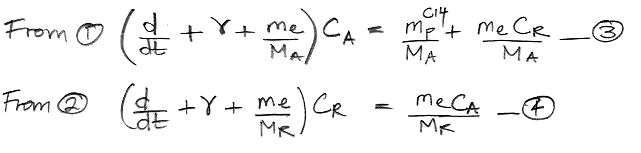The only variables in these two equations are CA, CR, and time, t. All other parameters are constants that we have already calculated. We must eliminate CR from the (3) so as to obtain an equation in CA and t alone, which we can then solve. We eliminate CR by multiplying (3) by the same factor that we observe on the left side of (4).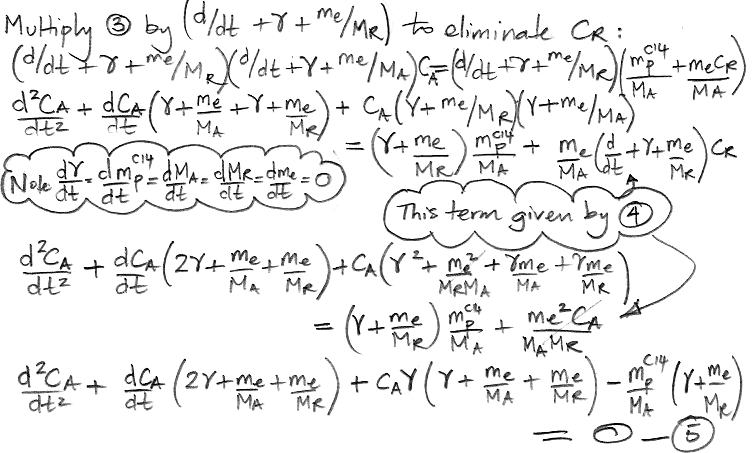We do the same thing for CR, arriving at a differential equation in only CR and t.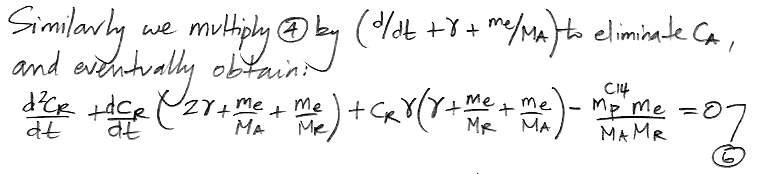At this point we pause to check the equations by considering how they behave as time approaches infinity, as shown here, and we find that they appear to behave correctly. Both equations have solutions of the same form: a constant plus two decay terms.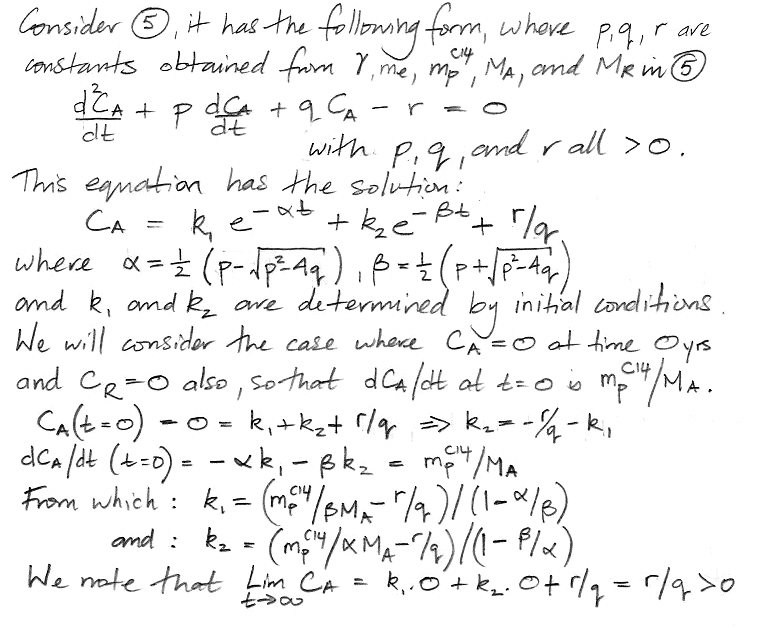We insert values for mpC14, γ, MA, MR, and me and obtain values for all five constants in our solution. The coefficients α and β dictate how rapidly the concentration evolves with time. We have α = 0.0574 and β = 0.000122. We see that α is close to the fraction of the atmospheric carbon that is exchanged with the reservoir every year, while β is close to the decay rate of carbon-14. We have 1/α = 17 yr, which is the time constant of exchanges between the atmosphere and the reservoir, and 1/β = 8,200 yrs, which is the time constant of accumulation of carbon-14 in the reservoir. The weighting factors k1 and k2 are −0.2 and −0.8 respectively. Together, they add up to −1.0 ppt. The constant term is the equilibrium value of CA, which comes out as 1.0 ppt, which is what we expect, because we chose the value of me and MR to make sure that the equilibrium concentration would be 1.0 ppt. Our equation for CA is as follows, where concentration is in ppt and time is in years.

CA = 1.0 − 0.2 et/17 − 0.8 et/8200.

At t = 0, we have CA = 0 ppt, and when t→∞, CA→1.0 ppt, as we expect. The 17-yr decay term represents the flow of carbon-14 into the reservoir. The 8200-yr term represents the accumulation of carbon-14 in the reservoir. We obtain a similar solution for CR.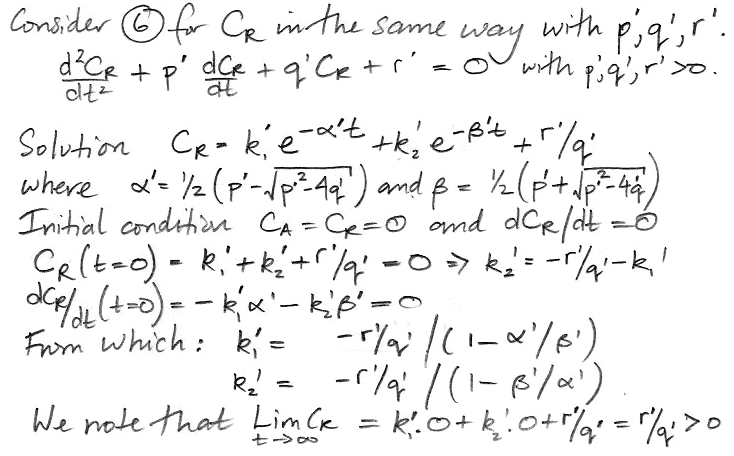The coefficients α' and β' are the same as α and β. But the weighting factors are different, as is the constant term. Our equation for CR is,

CR = 0.8 + 0.002 et/17 − 0.802 et/8200.

At t = 0, we have CR = 0 ppt, and dCR/dt = k1α+k2β = 0.0 ppt/yr, and d2CR/dt2 = k1α2+k2β2 > 0, all of which we expect, and also when t→∞, we have CR→0.8 ppt, which is one of our starting assumptions.

We are pleased to have an analytic solution for CA and CR. A numerical solution to the differential equations turns out to be unstable for time steps greater than ten years. We want to plot CA and CR over a hundred thousand years. Ten thousand steps are cumbersome in a spreadsheet. Furthermore, the analytic solution us gives more insight into the way the parameters of the carbon cycle interact to govern its behavior.